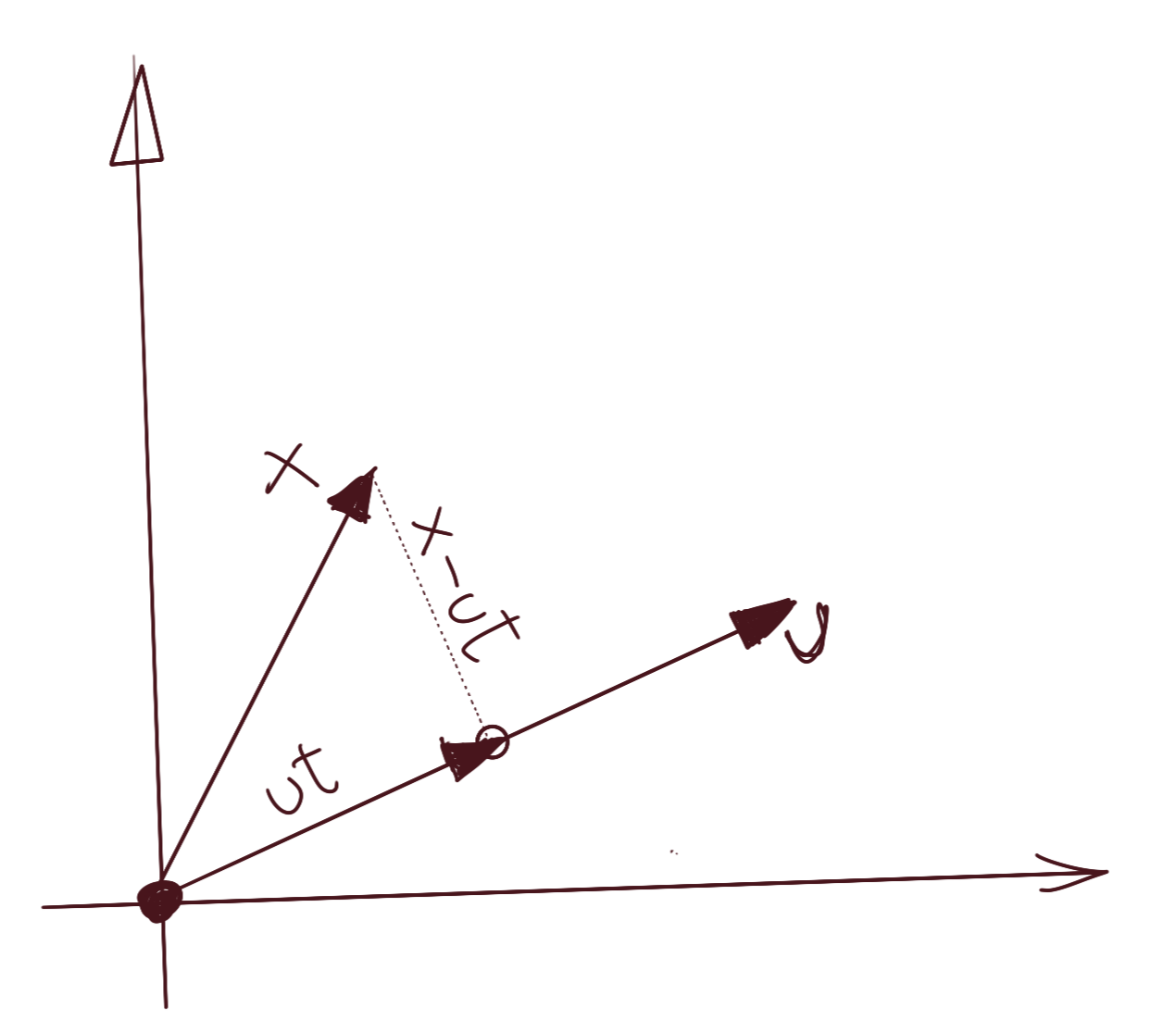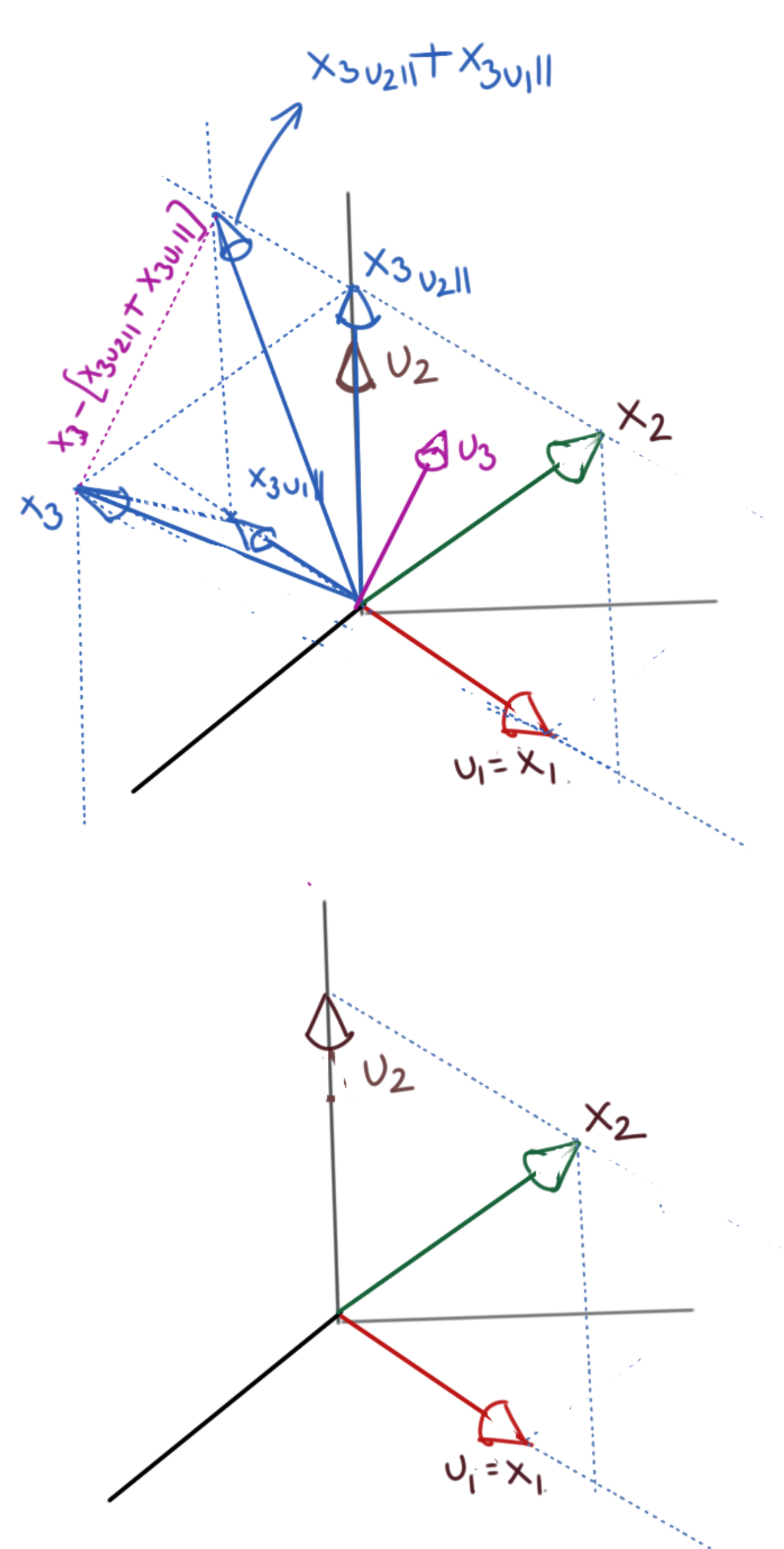# Total Internal Reflection

Technology and Art

# The Gram-Schmidt Orthogonalisation

Avishek Sen Gupta on 27 May 2021

We discuss an important factorisation of a matrix, which allows us to convert a linearly independent but non-orthogonal basis to a linearly independent orthonormal basis. This uses a procedure which iteratively extracts vectors which are orthonormal to the previously-extracted vectors, to ultimately define the orthonormal basis. This is called the Gram-Schmidt Orthogonalisation, and we will also show a proof for this.

## Projection of Vectors onto Vectors

This section derives the decomposition of a vector into two orthogonal components. These orthogonal components aren’t necessarily the standard basis vectors ($$\text{[1 0]}$$ and $$\text{[0 1]}$$ in $$\mathbb{R}^2$$, for example); but they are guaranteed to be orthogonal to each other.

Assume we have the vector $$\vec{x}$$ that we wish to decompose into two orthogonal components. Let us choose an arbitrary vector $$\vec{u}$$ as one of the components; we will derive its orthogonal counterpart as part of this derivation.Since the projection will be collinear with $$\vec{u}$$, let us assume the projection is $$t\vec{u}$$, where $$t$$ is a scalar. The only constraint we wish to express is that the vector $$\vec{u}$$ and the plumb line from the tip of the vector $$\vec{x}$$ to $$\vec{u}$$ are perpendicular, i.e., their dot product is zero. We can see from the above diagram that the plumb line is $$\vec{x}-t\vec{u}$$. We can then write:

$u^T.(x-ut)=0 \\ \Rightarrow u^Tx=u^Tut \\ \Rightarrow t={(u^Tu)}^{-1}u^Tx$

We know that $$u^Tu$$ is the dot product of $$\vec{u}$$ with itself, and thus a scalar, so you could write it as:

$t=\frac{u^Tx}{u^Tu}$

and indeed, we’d be justified in doing that, but let’s not make that simplification, because there is a more general case coming up, where this will not be a scalar. Thus, the component of $$\vec{x}$$ in the direction $$\vec{u}$$ is $$ut={(u^Tu)}^{-1}u^Txu$$ and the orthogonal component will be $$x-ut=x-{(u^Tu)}^{-1}u^Txu$$.

The one important thing to note is the expression for $$t$$ in the general case, i.e., when it is not a scalar. It is basically the expression for the left inverse of a general matrix:

There is one simplifying assumption we can make: if $$\vec{u}$$ is a unit vector, then $$u^Tu=I$$, which simplifies the expressions to:

$\mathbf{x_{u\parallel}={(u^Tu)}^{-1}u^Txu} \\ x_{u\parallel}=u^Txu \text{ (if u is a unit vector)}\\ \mathbf{x_{u\perp}=x-{(u^Tu)}^{-1}u^Txu} \\ x_{u\perp}=x-u^Txu \text{ (if u is a unit vector)}$

## Projection of Vectors onto Vector Subspaces

The same logic applies when we are projecting vectors onto vector subspaces. We use the same constraint, i.e.:

$u^T.(x-ut)=0$

There are a few differences in the meaning of the symbols worth noting. $$u$$ is no longer a single column vector; it is a set of column vectors which define a vector subspace. Let’s assume the vector subspace is embedded in $$\mathbb{R}^n$$, and we have $$m$$ linearly independent vectors in $$u$$ ($$m\leq n$$). $$u$$ now becomes a $$n\times m$$ matrix.

The projection is no longer gotten from scaling a single vector; it is now expressible as a linear combination of these $$m$$ vectors. This set of weightings is $$t$$, which now becomes a $$m\times 1$$ matrix. This change of $$t$$ from a scalar to a $$m\times 1$$ matrix is also the reason we didn’t simplify the $$u^Tu$$ expression in the previous section; in the general case, $$t$$ is not a scalar.

$$\vec{x}$$ is still an $$n\times 1$$ matrix; this hasn’t changed.

Thus, the results of projection of a vector onto a vector subspace are still the same.

$\mathbf{x_{u\parallel}={(u^Tu)}^{-1}u^Txu} \\ x_{u\parallel}=u^Txu \text{ (if u is a unit vector)}\\ \mathbf{x_{u\perp}=x-{(u^Tu)}^{-1}u^Txu} \\ x_{u\perp}=x-u^Txu \text{ (if u is a unit vector)}$ $t={(u^Tu)}^{-1}u^Tx$

## Gram-Schmidt Orthogonalisation

We are now in a position to describe the intuition behind Gram-Schmidt Orthogonalisation. Let us state the key idea first.

For a set of $$m$$ linearly independent vectors in $$\mathbb{R}^n$$ which span some subspace $$V_m$$, there exists aset of $$m$$ orthonormal basis vectors, which span the same subspace $$V_m$$.

The procedure goes as follows:

Assume $$m$$ linearly independent (but not orthogonal) vectors in $$\mathbb{R}^n$$. They span some subspace $$V_m$$ of dimensionality $$m$$. Let these vectors be $$x_1$$, $$x_2$$, $$x_3$$, …, $$x_m$$.

• We have to start somewhere, so let’s assume that our first orthogonal basis vector is $$u_1=\frac{x_1}{\|x_1\|}$$ (normalise to be a unit vector). $$u_1$$ is our first orthogonal basis vector.
• We now project $$x_2$$ onto $$u_1$$, finding $${x_2}_{u_1\parallel}$$ and $${x_2}_{u_1\perp}$$ as we have described in the previous sections. We won’t really use $${x_2}_{u_1\parallel}$$ except to calculate its orthogonal component $${x_2}_{u_1\perp}$$.
• Designate $$u_2={x_2}_{u_1\perp}$$. Because of the way we have constructed $$u_2$$, $$u_2$$ is orthogonal to $$u_1$$. We now have two orthogonal basis vectors, $$u_1$$, $$u_2$$. Normalise them to unit vectors as needed. Computationally, $$u_2$$ looks like this:

$u_2=x_2-{u_1}^Tx_{2}u_1$
• Now let us project $$x_3$$ onto $$u_1$$ and $$u_2$$ to get $$({x_3}_{u_1\parallel}, {x_3}_{u_2\parallel})$$. Calculate $${x_3}_{u_1,u_2\perp}=x_3-{x_3}_{u_1\parallel}-{x_3}_{u_2\parallel}$$.
• Designate $$u_3={x_3}_{u_1,u_2\perp}$$. We now have three orthogonal basis vectors, $$u_1$$, $$u_2$$, $$u_3$$. Normalise them to unit vectors as needed. Computationally, $$u_3$$ looks like this:

$u_2=x_3-{u_1}^Tx_{3}u_1-{u_2}^Tx_{3}u_2$
• Repeat the above procedure for all the remaining vectors upto $$x_m$$. At the end, we will have $$m$$ orthogonal basis vectors $$(u_1, u_2, ..., u_m)$$ which will span the same vector subspace $$V_m$$.You will notice that at every stage of this procedure, the next orthogonal basis vector to be computed, is given by the following general identity:

$u_{k+1}=x_{k+1}-\sum_{i=1}^{k}{u_i}^Tx_{k+1}u_i$

It is very easy to see that at every step, the latest basis vector is orthogonal to every other previously-generated basis vector. To see this, take the dot product on both sides with an arbitrary $$u_j$$, such that $$j\leq k$$.

${u_j}^Tu_{k+1}={u_j}^Tx_{k+1}-\sum_{i=1}^{k}{u_j}^T\underbrace{ ({u_i}^Tx_{k+1}) }_{scalar}u_i \\ ={u_j}^Tx_{k+1}-\sum_{i=1}^{k}\underbrace{ ({u_i}^Tx_{k+1}) }_{scalar}{u_j}^Tu_i$

Because of the way we have constructed the previous orthogonal basis vectors, we have $${u_j}^Tu_i=0$$ for all $$j\neq i$$, and $${u_j}^Tu_i=1$$ for $$j=i$$ (assuming unit basis vectors). Thus, the above identity becomes:

${u_j}^Tu_{k+1}={u_j}^Tx_{k+1}-{u_j}^Tx_{k+1}=0$

## Proof of Gram-Schmidt Orthogonalisation

A very valid question is: why does the basis from the Gram-Schmidt procedure span the same vector subspace as the one spanned by the original non-orthogonal basis?

The proof should make this clear; most of it follows almost directly from the procedure itself; we only need to fill in a few gaps, and formalise the presentation.

Given a set of $$m$$ linearly independent vectors $$(x_1, x_2, x_3, ..., x_m)$$ in $$\mathbb{R}^n$$ spanning a vector subspace $$V\in\mathbb{R}^m$$, there exists an orthogonal basis $$(u_1, u_2, u_3, ..., u_m)$$ which spans the vector subspace $$V\in\mathbb{R}^m$$.

We prove this by induction.

### 1. Proof for $$n=1$$

Let us validate the hypothesis for $$n=1$$. For $$x_1$$, if we take $$u_1=\frac{x_1}{\|x_1\|}$$, we can see that $$u_1$$ spans the same vector subspacee as $$x_1$$, since it’s merely a scaled version of $$x_1$$.

### 2. Proof for $$n=k+1$$

Let us now assume that the above statement holds for $$n=k\leq m$$, i.e., there are $$k$$ orthogonal basis vectors $$(u_1, u_2, u_3, ..., u_k)$$ which span the same vector subspace $$V\in\mathbb{R}^k$$ as the set $$(x_1, x_2, x_3, ..., x_k)$$.

Now, consider the construction of the $$(k+1)$$th orthogonal basis vector $$u_{k+1}$$ like so:

$u_{k+1}=x_{k+1}-\sum_{i=1}^{k}{u_i}^Tx_{k+1}u_i$

It is very easy to see that at every step, the latest basis vector is orthogonal to every other previously-generated basis vector. To see this, take the dot product on both sides with an arbitrary $$u_j$$, such that $$j\leq k$$.

${u_j}^Tu_{k+1}={u_j}^Tx_{k+1}-\sum_{i=1}^{k}{u_j}^T\underbrace{ ({u_i}^Tx_{k+1}) }_{scalar}u_i \\ ={u_j}^Tx_{k+1}-\sum_{i=1}^{k}\underbrace{ ({u_i}^Tx_{k+1}) }_{scalar}{u_j}^Tu_i$

Because of the way we have constructed the previous orthogonal basis vectors, we have $${u_j}^Tu_i=0$$ for all $$j\neq i$$, and $${u_j}^Tu_i=1$$ for $$j=i$$ (assuming unit basis vectors). Thus, the above identity becomes:

${u_j}^Tu_{k+1}={u_j}^Tx_{k+1}-{u_j}^Tx_{k+1}=0$

Thus, the newly constructed basis vector is orthogonal to every basis vector $$(u_1, u_2, u_3, ..., u_k)$$. This completes the induction part of the proof.

### 3. Proof that $$u_{k+1}\neq 0$$

We also prove that the newly-constructed basis vector is not a zero vector. For that, let us assume that $$u_{k+1}=0$$. Then, we get:

$x_{k+1}-\sum_{i=1}^{k}{u_i}^Tx_{k+1}u_i=0 \\ x_{k+1}=\sum_{i=1}^{k}{u_i}^Tx_{k+1}u_i$

This implies that $$x_{k+1}$$ is expressible as a linear combination of the set of vectors $$(u_1, u_2, u_3, ..., u_k)$$. But we have also assumed that this set spans the same vector subspace as $$(x_1, x_2, x_3, ..., x_k)$$.

This implies that $$x_{k+1}$$ is expressible as a linear combination of the set $$(x_1, x_2, x_3, ..., x_k)$$, which is a contradiction, since the vectors in the full set $$(x_1, x_2, x_3, ..., x_m)$$ are linearly independent. Thus, $$u_{k+1}$$ cannot be zero.

$\blacksquare$
tags: Machine Learning - Linear Algebra - Proofs - Theory